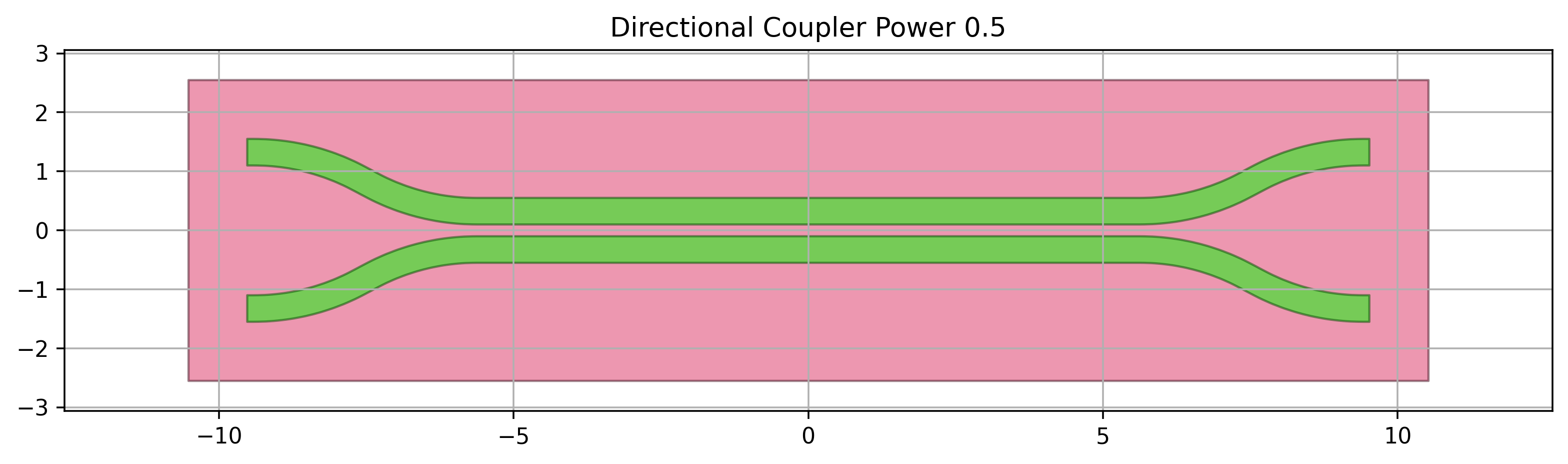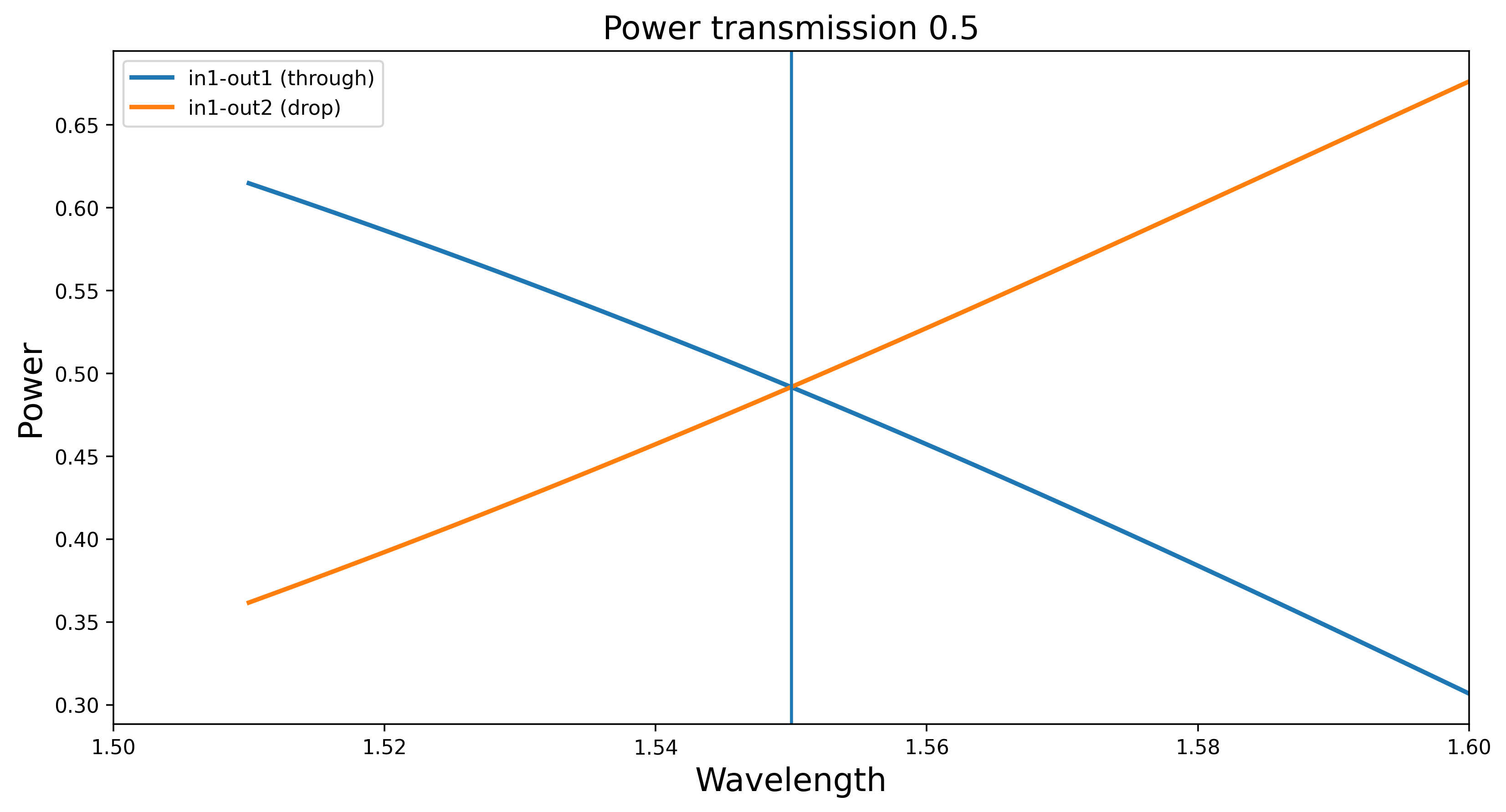# 1.1. MZI lattice filter¶

In this section, we will explain how to design a lattice filter based on Mach-Zender interferometers (MZI).

## 1.1.1. Directional coupler¶

The basic building block of an MZI is the directional coupler. In this case, we use DirectionalCouplerSPower from SiFab, the demonstration pdk distributed with this training material. This class is useful as it has a precomputed model, which is used to calculate the correct length of the directional coupler for a given power coupling coefficient into the cross arm. We can visualize this component, run a circuit simulation and plot the transmission. For more info on directional couplers available in SiFab, have a look at Directional coupler.

from si_fab import all as pdk
import numpy as np
import pylab as plt

dc = pdk.DirectionalCouplerSPower(power_fraction=0.5,
target_wavelength=1.55)
dc_lv = dc.Layout()
dc_lv.visualize(annotate=True)

dc_cm = dc.CircuitModel()

wavelengths = np.linspace(1.51, 1.6, 500)
S = dc_cm.get_smatrix(wavelengths=wavelengths)
plt.figure()
plt.plot(wavelengths, np.abs(S["in1", "out1"])**2, linewidth=2.2, label="in1-out1 (through)")
plt.plot(wavelengths, np.abs(S["in1", "out2"])**2, linewidth=2.2, label="in1-out2 (drop)")
plt.axvline(x=dc.target_wavelength)
plt.title("Power transmission", fontsize=16)
plt.xlabel("Wavelength", fontsize=16)
plt.ylabel("Power", fontsize=16)
plt.xlim(1.5, 1.6)
plt.legend(fontsize=14, loc=1)
plt.legend()
plt.show()## 1.1.2. MZI lattice filter¶

Now we can use this directional coupler to build an MZI lattice filter. To achieve this, we define a Python class that inherits from CircuitCell and we call it MZILatticeFilter. This lattice filter is a parametric circuit with customizable number of cascaded MZIs. The lengths of the directional couplers used for the MZIs are automatically calculated by providing a list of power coupling coefficients.

class MZILatticeFilter(CircuitCell):
""" Mach-Zender interferometer lattice filter based on directional couplers with different power
coupling.
The number of power couplings should be equal to the number of delay lengths plus 1.
"""
directional_couplers = i3.ChildCellListProperty(doc="list of directional couplers")
center_wavelength = i3.PositiveNumberProperty(default=1.55, doc="Center wavelength")
delay_lengths = i3.ListProperty(default=[100.], doc="List of delay lengths")
phase_error_width_deviation = i3.NonNegativeNumberProperty(default=0.0)
phase_error_correlation_length = i3.NonNegativeNumberProperty(default=0.0)

def _default_directional_couplers(self):
power_couplings = [0.5, 0.5]
dir_couplers = [pdk.DirectionalCouplerSPower(name=self.name + "dc_{}".format(cnt),
power_fraction=p,
target_wavelength=self.center_wavelength)
for cnt, p in enumerate(power_couplings)]
return dir_couplers

def get_connector(self, offset_high):
from circuit.connector_functions import get_template

def connector(start_port, end_port, name=None, shape=None, connector_kwargs={}):
tt = get_template(start_port, end_port)
tt_new = tt.cell.modified_copy()
tt_new.CircuitModel(phase_error_width_deviation=self.phase_error_width_deviation,
phase_error_correlation_length=self.phase_error_correlation_length)
shape = [
start_port.position,
start_port.position + (min_straight, 0.0),
start_port.position + (min_straight, offset_high),
end_port.position + (-min_straight, offset_high),
end_port.position - (min_straight, 0.0),
end_port.position,
]
cell = i3.RoundedWaveguide(name=name, trace_template=tt_new)
return cell

return connector

def _default_child_cells(self):
child_cells = dict()
for cnt, dc in enumerate(self.directional_couplers):
child_cells["dc_{}".format(cnt)] = dc
return child_cells

def _default_connectors(self):
conn = []
for cnt, delay_length in enumerate(self.delay_lengths):
if delay_length > 0:
l_top = delay_length / 2
l_bot = 0
else:
l_top = 0
l_bot = - delay_length / 2
p1 = "dc_{}:out1".format(cnt)
p2 = "dc_{}:in1".format(cnt + 1)
conn.append((p1, p2, self.get_connector(offset_high=- 2 * self.bend_radius - l_bot)))
p1 = "dc_{}:out2".format(cnt)
p2 = "dc_{}:in2".format(cnt + 1)
conn.append((p1, p2, self.get_connector(offset_high=2 * self.bend_radius + l_top)))
return conn

def _default_place_specs(self):
place_specs = []
for cnt in range(1, len(self.directional_couplers)):
spec = i3.PlaceRelative("dc_{}:in1".format(cnt), "dc_{}:out1".format(cnt - 1), (distance, 0))
place_specs.append(spec)
return place_specs

def _default_external_port_names(self):
epn = {"dc_0:in1": "in1",
"dc_0:in2": "in2",
"dc_{}:out1".format(len(self.delay_lengths)): "out1",
"dc_{}:out2".format(len(self.delay_lengths)): "out2"}
return epn


Let’s analyze the code above:

1. Properties. We defined a list of properties that are used to design the MZI lattice filters. The length of the list provided in power_couplings determines how many directional couplers are used to design the lattice filter. If 5 values are provided for the power coupling coefficients, then 4 values should be provided for the delay_lengths to be used to connect the 5 directional couplers to each other. The phase error properties can be used to perform a variability analysis of the MZI lattice filter.
2. Default directional couplers. In _default_directional_couplers we instantiate as many directional couplers as provided in power_couplings. As mentioned earlier, the default directional coupler used in this example is DirectionalCouplerSPower from SiFab.
3. get_connector function. This function returns a connector which is used to draw the arms connecting the directional couplers to each other.
4. CircuitCell properties. Next, the properties needed to create a circuit with CircuitCell are specified:
1. The directional couplers are added to the dictionary of child cells in _default_child_cells.
2. The connectors between the directional couplers are specified in _default_connectors. The function get_connector is used inside this method to obtain arms with length given by the values in delay_lengths.
3. The placement of the directional couplers is defined in _default_place_specs.
4. The external ports of the final MZI lattice filter are exposed in _default_external_port_names.

The connectivity defined in CircuitCell is automatically used to extract the netlist of the circuit and to perform circuit simulations.